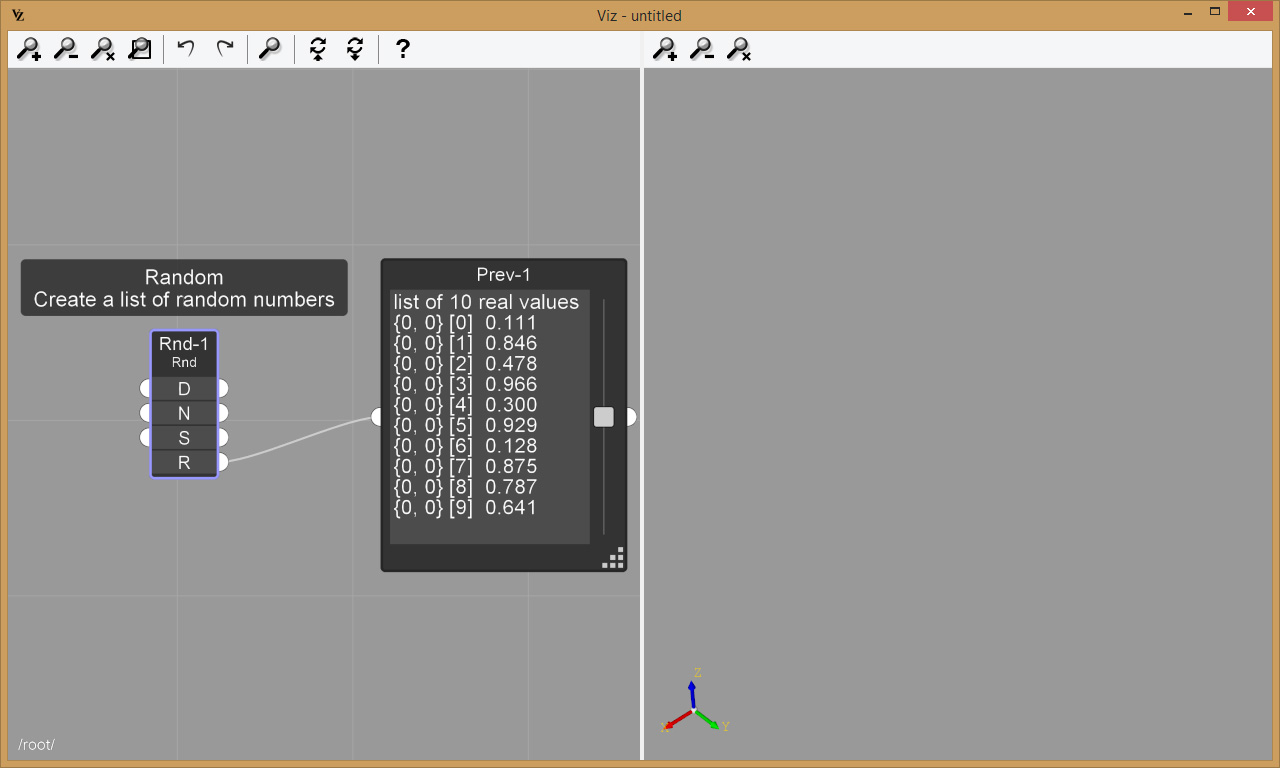## Viz Pro Manual

### Lists

Many nodes (Random, Range, Series, etc.) generate multiple output data for each single input data. This output data is represented in Viz as a list. In the example below, we have a Random node, which generate a list of random numbers:In the example above, we used a Preview node to preview the generated list of 10 random numbers. Looking a the preview node, the numbers between square brackets [] represent the index in the list. For now, let’s ignore the element {0, 0}, which will be needed when using multi-dimensional lists (multilists).

Next: Creating an array of cylinders